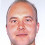# Learning Clojure

## Monday, May 12, 2014

### Planes in Space : Checking by Hand

```;; Ok, so I reckon:

(defn regions [n m]
(cond (= n 1) (list m (inc m))
(= m 0) (concat (repeat n 0) '(1))
:else (map +
(regions n (dec m))
(concat '(0) (regions (dec n) (dec m)))
(concat (regions (dec n) (dec m)) '(0)))))

;; In fact, I reckon that I can prove it.

;; It's quite easy to prove something that isn't true. Usually when
;; you run across a counterexample, that shows you where the
;; unsuspected false step in your reasoning was.

;; So I'd like to verify the formula on lots of examples.

;; But how?

;; These I can count in my head.

(regions 3 0) ;-> (0 0 0 1) ; just space, no planes
(regions 3 1) ;-> (0 0 1 2) ; a plane cuts space in half
(regions 3 2) ;-> (0 1 4 4) ; two planes intersect in one line, cutting space into four
(regions 3 3) ;-> (1 6 12 8) ; 1 point where three planes meet,
; six coordinate half-axes,
; three coordinate planes divided into four quadrants each,
; and eight octants

;; I am sort of confident that four planes make fifteen regions and intersect in four points.
(regions 3 4) ;-> (4 18 28 15)
;; But every attempt to count the 18 lines and 28 regions I make ends up relying on
;; the sort of arguments I made to make the recursion in the first place.

;; And at this point my intuition breaks.
(regions 3 5) ;-> (10 40 55 26)

;; I mean, five planes, any three intersect in a point,
;; 10 ways to choose 3 planes from five, so 10 points,
;; but after that I'm dead.

;; And this? Six choose 3 is 20, I can see that....
(regions 3 6) ;-> (20 75 96 42)

;; And I defy anyone to even picture
(regions 5 8) ;-> (56 350 896 1176 792 219)

;; 8 choose 5 is (/ (* 8 7 6) (* 1 2 3)) = 56, which is fair enough.

;; 8 choose 4 is (/ (* 8 7 6 5) (* 1 2 3 4)) is 70

;; If we take any 4 hyperplanes from our 8, they'll define a line.
;; The four remaining hyperplanes then divide each line like:
(regions 1 4) ;-> (4 5)
;; So if each of those lines is sliced into 5 pieces then that's our 350.
;; But I'm just using the same recursive argument again.

;; So I don't even know if that's evidence or not.

;; One nice thing about it, the formula has an alternating sum property,
;; like the Euler index.

(defn signature [lst]
(reduce +
(map *
(apply concat (repeat '(+1 -1)))
(reverse lst))))

(signature (regions 5 8)) ;-> 1

(def regions (memoize regions))
(regions 7 23) ;-> (245157 1817046 5787628 10271800 10973116 7057688 2531288 390656)
(signature (regions 7 23)) ;-> 1

(regions 23 20) ;-> (0 0 0 1 40 760 9120 77520 496128 2480640 9922560 32248320 85995520 189190144 343982080 515973120 635043840 635043840 508035072 317521920 149422080 49807360 10485760 1048576)
(signature (regions 23 20)) ;-> 1

;; But annoyingly, you can just read that property straight from the recursion!
```

1.2.